# Absolute or Relative

When you refer to cells you have two options.
You can either refer to cells using Relative References or you can use Absolute References.
Relative References will change so they refer to cells relative to the cell containing the formula.
Absolute References will always refer to the same cells.
Most worksheets contain formulas so it is very important to understand the difference between the two.

 A1 Relative cell reference \$A\$1 Absolute cell reference \$A1 Column absolute cell reference A\$1 Row absolute cell reference

You can change the type of cell reference by pressing F4 to toggle between the four different types of cell reference.

### Relative References

This is the easiest type of reference to understand and is the one used when you use the mouse to select cells in your formulas.
No change when you cut and paste - Makes a change though when you copy and paste

Relative formulas are displayed as a column letter combined with a row number (for example: B2, D10, F35, etc).
For very simple worksheets and formulas using this type of cell reference is exactly what you want to use.
When you use relative references you can easily copy formulas down (or across) when you are working with tables of data.
Lets imagine the following table and lets assume that we want to include a total at the end of each row and underneath each column.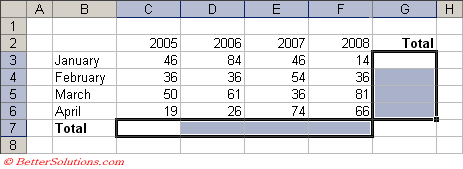Select cell "G3" and enter the following "=SUM("
You can then use the mouse to select cells "C3:F3" and press Enter to insert the total.
Select cell "G3" and use the handle in the bottom right corner to drag the cell down to cell "G6".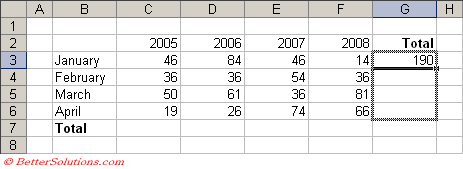You will see that the formulas have been automatically adjusted so they are relative to each row in the table.
Using relative cell references allows you to quickly drag a formula and have it automatically adjust relative to the cell containing the formula.
You can repeat this for the totals at the bottom. Select cell "C7" and enter the following "=SUM(".
This time instead of using the mouse, type the cell reference "C3:C6" straight into the formula bar followed by a ")".
Press Enter and drag this cell across to cell "F7" to display all the column totals.

### Absolute References

There may be times when you want to use a "fixed" cell reference rather than a relative cell reference.
Absolute cell references are displayed with a dollar sign before the column letter and a dollar sign before the row number (for example \$B\$2, \$D\$10, \$F\$35, etc)
One example where an absolute (or fixed) cell reference might be needed is when you want to refer to a constant value in your formulas.
Lets image the following table and lets assume that we want to include a total at the end of each week showing the total amount of money earned.
This formula will need to be the total number of the hours worked that week multiplied by the hourly rate.
Select cell "H5" and enter the following formula.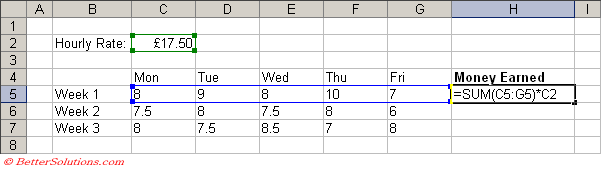Dragging this formula down to cell "H7" will not generate the correct formulas in cells "H6" and "H7".
Before we drag this formula down we need to "fix" the cell reference "C2" so this is not automatically adjusted.
We can change the C2 relative reference to an absolute cell reference by inserting dollar signs infront of the C and the 2.
Once we have changes the cell reference to absolute we can drag the formula knowing that every row will be referring to cell "C2".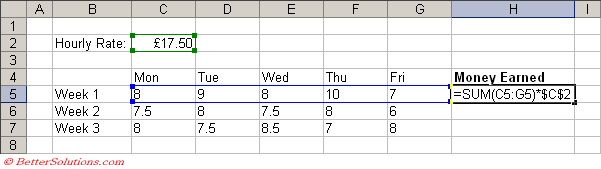Press Enter and drag this cell down to cell "H7" to display all the weekly totals.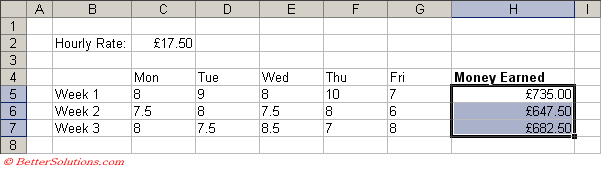### Mixed References

It is also possible to have "Mixed References".
Row Absolute ( =A\$1 ) - Relative column reference and Absolute row reference. The row number always remains the same but the column changes.
Column Absolute ( =\$A1 ) - Absolute column reference and Relative row reference. The column letter always remains the same but the row number changes.

### Using F4

You can press the F4 key multiple times when the cursor is in a cell reference to toggle between the different types. This works in both the formula bar and in cells directly.

### Copying Absolute References

Select cells(s), (Edit > Replace)
Find What - "="
Replace with - "@"
Replace All
Copy the necessary formulas, then Replace Back

### Important

The (F4) shortcut key toggles between the four different types of references.
When you cut and paste formulas containing Relative references, the references are NOT adjusted automatically.